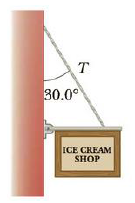Chapter 8, Problem 25P

Chapter
Section
Textbook Problem

A 500.-N uniform rectangular sign 4.00 m wide and 3.00 m high is suspended from a horizontal, 6.00-m-long, uniform, 100.-N rod as indicated in Figure P8.25. The left end of the rod is supported by a hinge, and the right end is supported by a thin cable making a 30.0° angle with the vertical, (a) Find the tension T in the cable, (b) Find the horizontal and vertical components of force exerted on the left end of the rod by the hinge.Figure P8.25

(a)

To determine
The tension in the cable.

Explanation

Given info: The weight of the sign board is 500N , length of the board is 4.00m , length of the rod is 6.00m , and the weight of the rod is 100N .

Explanation: The vertical component of the tension in the cable can be determined by the conditions τ=0 such that L(Tycosθ)(L/2)WR(LL'/2)Ws=0 and Ty=Tcosθ .

The following figure shows the forces involved in rod-sign board system.

The formula for the tension in the cable is,

T=(WR/2)+(1L'/2L)WBcosθ

• WR is weight of the rod.
• WB is weight of the sign

(b)

To determine
The horizontal and vertical components of force exerted on the left end of the rod by the hinge.

Still sussing out bartleby?

Check out a sample textbook solution.

See a sample solution

The Solution to Your Study Problems

Bartleby provides explanations to thousands of textbook problems written by our experts, many with advanced degrees!

Get Started

What makes up a system of units?

An Introduction to Physical Science

Who has more inertia, a child sitting on the sofa or a heavy man jogging?

Physics for Scientists and Engineers: Foundations and Connections

Can you suggest any ways humans might be altering biogeochemical cycles?

Oceanography: An Invitation To Marine Science, Loose-leaf Versin